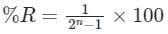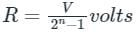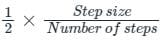Test: Data Converters - 2

# Test: Data Converters - 2

Test Description

## 10 Questions MCQ Test Topicwise Question Bank for Electronics Engineering | Test: Data Converters - 2

Test: Data Converters - 2 for Electronics and Communication Engineering (ECE) 2023 is part of Topicwise Question Bank for Electronics Engineering preparation. The Test: Data Converters - 2 questions and answers have been prepared according to the Electronics and Communication Engineering (ECE) exam syllabus.The Test: Data Converters - 2 MCQs are made for Electronics and Communication Engineering (ECE) 2023 Exam. Find important definitions, questions, notes, meanings, examples, exercises, MCQs and online tests for Test: Data Converters - 2 below.
Solutions of Test: Data Converters - 2 questions in English are available as part of our Topicwise Question Bank for Electronics Engineering for Electronics and Communication Engineering (ECE) & Test: Data Converters - 2 solutions in Hindi for Topicwise Question Bank for Electronics Engineering course. Download more important topics, notes, lectures and mock test series for Electronics and Communication Engineering (ECE) Exam by signing up for free. Attempt Test: Data Converters - 2 | 10 questions in 30 minutes | Mock test for Electronics and Communication Engineering (ECE) preparation | Free important questions MCQ to study Topicwise Question Bank for Electronics Engineering for Electronics and Communication Engineering (ECE) Exam | Download free PDF with solutions
 1 Crore+ students have signed up on EduRev. Have you?
Test: Data Converters - 2 - Question 1

### The speed of conversion is maximum in

Detailed Solution for Test: Data Converters - 2 - Question 1

Flash ADC is also known as parallel comparative A/D converter

1) Flash  ADC - Faster speed and low noise immunity

2) SAC  ADC - Low noise immunity and medium speed

3) RAMP  ADC - Higher resolution and good noise immunity

4) Dual Slope  ADC - Low speed and good noise immunity

For n-bit conversion, the conversion time for different ADC are:

Counter type ADC: (2n – 1) Tclk

Successive approx. time ADC: n Tclk

Dual slope ADC: (2n+1 – 1) Tclk (Maximum time is taken)

Test: Data Converters - 2 - Question 2

### The minimum number of comparators required to build an 8 bit flash ADC

Detailed Solution for Test: Data Converters - 2 - Question 2

Concept:

2) An n-bit flash type ADC requires:

2n -1 comparators, 2n resistors and one 2n × n priority encoder.

Calculation:

The number of comparators required to build an 8-bit flash ADC will be:

N = 28 - 1

N = 256 - 1

N = 255

Test: Data Converters - 2 - Question 3

### The difference between analog voltage represented by two adjacent digital codes of an analog to digital converter is

Detailed Solution for Test: Data Converters - 2 - Question 3

Resolution: It is defined as the smallest change in the analog output voltage corresponding to a change of one bit in the digital output.

The percentage resolution (%R) of an n-bit DAC is:The resolution of an n-bit DAC with a range of output voltage from 0 to V is given by:Hence the difference between analog voltage represented by two adjacent digital codes of an analog to digital converter is called resolution.

Hence option (B) is the correct answer.

Test: Data Converters - 2 - Question 4

Maximum value of signal to noise ratio of an 8 bit ADC with an I/P range of 10 V will be

Detailed Solution for Test: Data Converters - 2 - Question 4

Concept:

For an n-bit ADC signal to noise ratio is given by

= 1.8 + 6 n dB

Where message signal → sinusoidal & noise PDF = uniform distribution

Calculation:

Given data n = 8 bit

Signal to noise ratio (SNR) = 1.8 + 6 n dB

= 1.8 + 6 x 8

= 49.8 dB

Test: Data Converters - 2 - Question 5

An analog voltage in the range 0 to 5V is divided into 10 equal intervals for conversion into 4 bit digital output. The maximum quantization error (in V) is -

Detailed Solution for Test: Data Converters - 2 - Question 5

Concept:

Maximum Quantization error =Step size= (Vmax - Vmin)

Calculation:

Given

Analog voltage ranging from 0 V (Vmin) to 5 V (Vmax)

No. of interval or number of steps = 10

Step size = (5 - 0) = 5 V

Maximum quantization error = 1/2 × (5/10) = 0.25 V

Test: Data Converters - 2 - Question 6

What is the output of a digital to analog converter?

Detailed Solution for Test: Data Converters - 2 - Question 6

The Digital to analog converter takes the binary number as an input and produces the analog voltage proportional to the binary number. These analog voltages represent specific analog voltage levels and have stairstep characters.

Test: Data Converters - 2 - Question 7

What is the error that occurs when the number of bits is not sufficient enough to represent the analog voltages?

Detailed Solution for Test: Data Converters - 2 - Question 7

When the number of bits is not sufficient enough to represent the analog voltage levels, quantization error occurs. The greater the number of bits, the greater the number of increments over the analog range and the smaller the quantizing error.

Test: Data Converters - 2 - Question 8

What is the minimum frequency of sampling so that the analog waveform is adequately expressed?

Detailed Solution for Test: Data Converters - 2 - Question 8

The minimum frequency at which an analog wave should be sampled is given by Nyquist frequency. If the sampling frequency is below this limit then the analog waveform is not fully represented and leads to data loss.

Test: Data Converters - 2 - Question 9

What is the main role of an ADC?

Detailed Solution for Test: Data Converters - 2 - Question 9

ADC corresponds to Analog to Digital Converter which is used to convert an analog signal into a digital. Digital signal can be processed and manipulated.

Test: Data Converters - 2 - Question 10

What is the signal frequency if the rectangular digital wave has a time period of 71.4μs?

Detailed Solution for Test: Data Converters - 2 - Question 10

Signal frequency = 1/ time period of the wave = 1/71.4μs = 14,006Hz.

## Topicwise Question Bank for Electronics Engineering

193 tests
Information about Test: Data Converters - 2 Page
In this test you can find the Exam questions for Test: Data Converters - 2 solved & explained in the simplest way possible. Besides giving Questions and answers for Test: Data Converters - 2, EduRev gives you an ample number of Online tests for practice

## Topicwise Question Bank for Electronics Engineering

193 tests(Scan QR code)